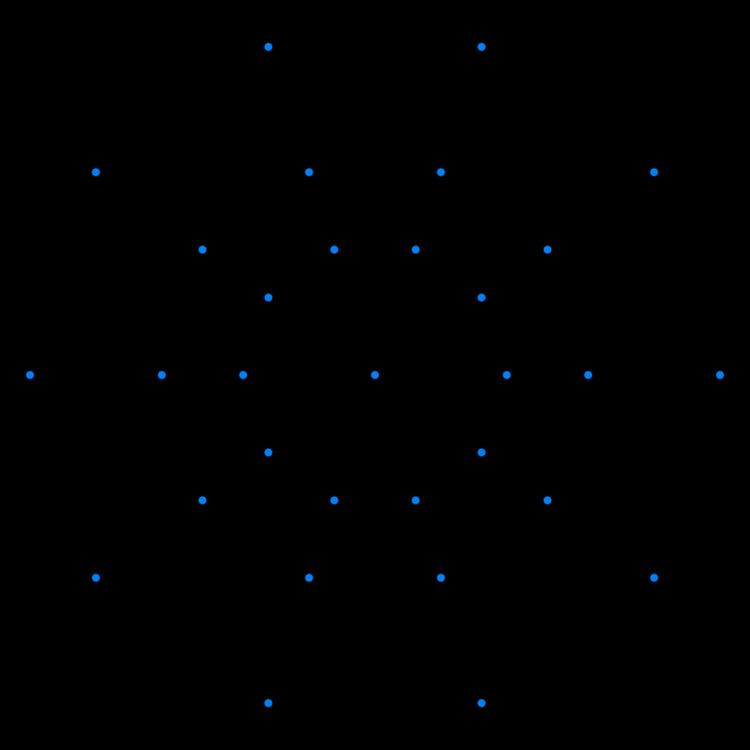# 6 demicube

Updated on
Covid-19In geometry, a 6-demicube or demihexteract is a uniform 6-polytope, constructed from a 6-cube (hexeract) with alternated vertices truncated. It is part of a dimensionally infinite family of uniform polytopes called demihypercubes.

## Contents

E. L. Elte identified it in 1912 as a semiregular polytope, labeling it as HM6 for a 6-dimensional half measure polytope.

Coxeter named this polytope as 131 from its Coxeter diagram, with a ring on one of the 1-length branches, . It can named similarly by a 3-dimensional exponential Schläfli symbol { 3 3 , 3 , 3 3 } or {3,33,1}.

## Cartesian coordinates

Cartesian coordinates for the vertices of a demihexeract centered at the origin are alternate halves of the hexeract:

(±1,±1,±1,±1,±1,±1)

with an odd number of plus signs.

There are 47 uniform polytopes with D6 symmetry, 31 are shared by the B6 symmetry, and 16 are unique:

The 6-demicube, 131 is third in a dimensional series of uniform polytopes, expressed by Coxeter as k31 series. The fifth figure is a Euclidean honeycomb, 331, and the final is a noncompact hyperbolic honeycomb, 431. Each progressive uniform polytope is constructed from the previous as its vertex figure.

It is also the second in a dimensional series of uniform polytopes and honeycombs, expressed by Coxeter as 13k series. The next figure is the Euclidean honeycomb 133 and the final is a noncompact hyperbolic honeycomb, 134.

6-demicube Wikipedia

Topics